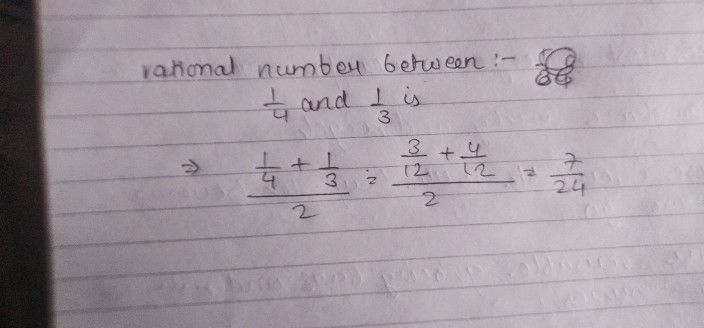Symbol
ProblemEXERCISE $1F$ $1.$ Find a rational number $e\pi xeen\dfrac {1} {4}$ and 3\ $2P$ Find a rational number between 2 and $3$ $3$ Find a rational number between $\dfrac {1} {3}$ and $\dfrac {1} {2}$ Find two rational numbers between $-3$ and $5$ Find three rational numbers between 4 and $2$ $45$ $4\dfrac {3} {4}$ $\dfrac {5} {6}$ 6. Find three rational numbers between $n\dfrac {2} {3}$ and $7.$ Find $10$ rational numbers between $\dfrac {-3} {4}$ and Find $12$ rational numbers between $-1$ and
7th-9th grade
Calculus
Search count: 108
SolutionQanda teacher - Ravi74please rate my answer 5 starsStudent
I do not understand
please answer the all question sir